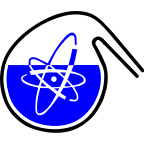#RadChem 2018

13-18 May 2018
Casino Conference Centre
Europe/Prague timezone

## $^{99}$Mo production from $\alpha$-induced reaction on $^{96}$Zr

16 May 2018, 09:15
15m
Red Hall (Casino Conference Centre)

### Red Hall

#### Casino Conference Centre

Reitenbergerova 4/95, Mariánské Lázně, Czech Republic
Verbal Production and Application of Radionuclides

### Speaker

Prof. Masayuki Aikawa (Hokkaido University)

### Description

Diagnostic imaging using $^{99m}$Tc ($T_{1/2}$ = 6.0 h), which is produced from the decay of $^{99}$Mo ($T_{1/2}$ = 66 h), is relying on the production of $^{99}$Mo from nuclear reactors to be shutdown. Alternative routes to produce the $^{99}$Mo and $^{99m}$Tc isotopes are being investigated. One of the reactions to create $^{99}$Mo is the $^{96}$Zr($\alpha$,n)$^{99}$Mo reaction. There are several experimental cross section datasets measured earlier, which are different from the TENDL data in the peak region of the excitation function of the reaction. To evaluate the production yield of the $^{99}$Mo isotope it is very important to provide reliable and consistent cross section data. Therefore, we performed two experiments to measure the cross sections the $^{96}$Zr($\alpha$,n)$^{99}$Mo reaction.

Two stacked targets were created from thin Zr foils with natural isotopic composition (10 $\mu$m and 20 $\mu$m) and Ti foils (5 $\mu$m) for monitoring the beam parameters. Both stacked targets were irradiated by 50 MeV alpha beam with intensity of about 200 pnA for 2 hours at the RIKEN AVF cyclotron. No chemical separation was applied. Cross sections of the $^{96}$Zr($\alpha$,n)$^{99}$Mo reaction were derived by measuring the $\gamma$ rays from decay of $^{99}$Mo using a high resolution, low background $\gamma$-spectrometer based on a HPGe detector.

The measured activation cross sections for formation of $^{99}$Mo are different from the available experimental data measured earlier in different studies. According to the newly measured excitation function the peak is located at around 14 MeV, which is lower than those for the literature data. On the contrary, the amplitude of the excitation function measured in this work is higher than those of the earlier studies.

### Primary authors

Mr Tomohiro Murata (Hokkaido University) Prof. Masayuki Aikawa (Hokkaido University) Ms Moemi Saito (Hokkaido University) Dr Naoyuki Ukon (Advanced Clinical Research Center, Fukushima Medical University) Dr Yukiko Komori (Nishina Center for Accelerator-Based Science, RIKEN) Dr Hiromitsu Haba (Nishina Center for Accelerator-Based Science, RIKEN) Dr Sándor Takács (Institute for Nuclear Research, Hungarian Academy of Sciences (ATOMKI))

### Presentation Materials

There are no materials yet.